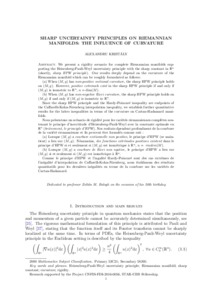REAL

Sharp Uncertainty Principles on Riemannian Manifolds: the influence of curvature

Kristály, Alexandru (2017) Sharp Uncertainty Principles on Riemannian Manifolds: the influence of curvature. Journal de Mathématiques Pures et Appliquées. ISSN 0021-7824 (In Press)Preview
Text
HPW-uncertainty-revised-Kristaly.pdf

We present a rigidity scenario for complete Riemannian manifolds supporting the Heisenberg-Pauli-Weyl uncertainty principle with the sharp constant in $\mathbb R^n$ (shortly, {\it sharp HPW principle}). Our results deeply depend on the curvature of the Riemannian manifold which can be roughly formulated as follows: (a) When $(M,g)$ has {\it non-positive sectional curvature}, the sharp HPW principle holds on $(M,g)$. However, {\it positive extremals exist} in the sharp HPW principle if and only if $(M,g)$ is isometric to $\mathbb R^n$, $n={\rm dim}(M)$. (b) When $(M,g)$ has {\it non-negative Ricci curvature}, the sharp HPW principle holds on $(M,g)$ if and only if $(M,g)$ is isometric to $\mathbb R^n$. Since the sharp HPW principle and the Hardy-Poincar\'e inequality are endpoints of the Caffarelli-Kohn-Nirenberg interpolation inequality, we establish further quantitative results for the latter inequalities in terms of the curvature on Cartan-Hadamard manifolds.Edit Item